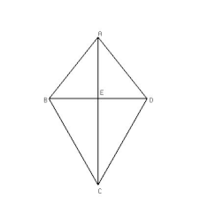# Proof by Transformation

#### burt

##### Junior Member
I was given the following question:

ABCD is a kite and point E is the intersection of its diagonals. Which of the following transformations in (a)-(d) can be used to prove that $$\displaystyle \triangle ABC \cong \triangle ADC$$ using the transformation definition of congruence?(a) The rotation around E of 180 degrees
(b) The translation from B to E
(c) The rotation around E of 90 degrees
(d) The reflection with respect to $$\displaystyle \overline{AC}$$

I'm not sure how to solve this. I know that if I reflect over the line AC, that will map the shape exactly to itself, but I don't know if that proves congruence. I also cannot figure out if any of the other ways can do this. Can anyone help me out a bit?

#### pka

##### Elite Member
ABCD is a kite and point E is the intersection of its diagonals. Which of the following transformations in (a)-(d) can be used to prove that $$\displaystyle \triangle ABC \cong \triangle ADC$$ using the transformation definition of congruence?
View attachment 20738
(a) The rotation around E of 180 degrees
(b) The translation from B to E
(c) The rotation around E of 90 degrees
(d) The reflection with respect to $$\displaystyle \overline{AC}$$
I'm not sure how to solve this. I know that if I reflect over the line AC, that will map the shape exactly to itself, but I don't know if that proves congruence. I also cannot figure out if any of the other ways can do this. Can anyone help me out a bit?
I do not have a definition of "transformation definition of congruence". My goto reference is James R Smart's Modern Geometries.
Nothing there. But there is a definition for $$\Delta ACB\cong \Delta ACD$$ means $$m(\angle ACB)=m(\angle ACD), m(\angle ABC)=m(\angle ABD)~\&~m(\angle CBA)=m(\angle CDA)$$
Moreover, $$\overline{AC}\cong\overline{AC},~\overline{AB}\cong\overline{AD}~\&~\overline{BC}\cong\overline{DC}$$ in that order.
Now a reflection across $$\overline{AC}$$ achieves that.
I hope that is of some use to you.

#### Dr.Peterson

##### Elite Member
I was given the following question:

ABCD is a kite and point E is the intersection of its diagonals. Which of the following transformations in (a)-(d) can be used to prove that $$\displaystyle \triangle ABC \cong \triangle ADC$$ using the transformation definition of congruence?

View attachment 20738
(a) The rotation around E of 180 degrees
(b) The translation from B to E
(c) The rotation around E of 90 degrees
(d) The reflection with respect to $$\displaystyle \overline{AC}$$

I'm not sure how to solve this. I know that if I reflect over the line AC, that will map the shape exactly to itself, but I don't know if that proves congruence. I also cannot figure out if any of the other ways can do this. Can anyone help me out a bit?
Can you state the "transformation definition of congruence" as it was given to you?

I would imagine it would be that if an isometry (which all the transformations mentioned are) transforms one figure to another [in this case, ABC to DBC], then they are congruent. That seems to be what you have seen, except that you only mentioned the entire "shape", not the two individual triangles you are asked about.

#### HallsofIvy

##### Elite Member
I would be inclined to suspect that the "Transformation Definition of Congruence" is simply that "two objects are congruent if and only if one can be transformed into the other". In that case, the fact that (d) transforms one triangle into the other is all you need!